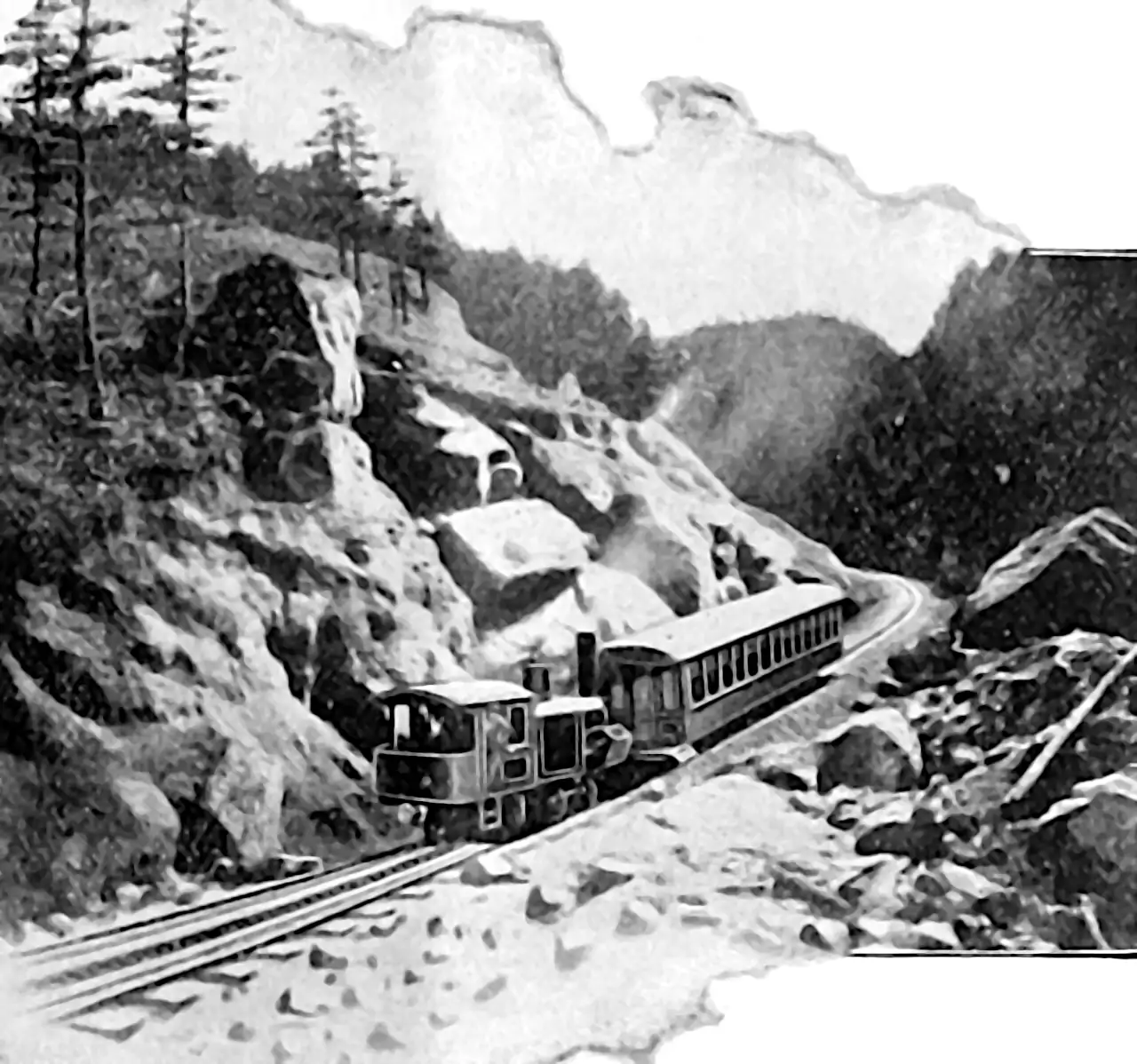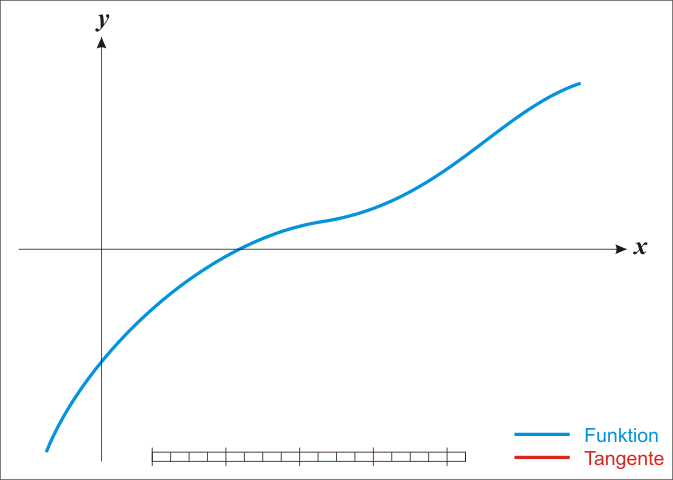NB ⚠️⛔️☣️: Under reconstruction; I think I need to characterise the 2nd-order Hessian approximations better.

Newton-type optimization, unlike basic gradient descent, uses (possibly approximate) 2nd-order gradient information to find the argument which minimises $x^*=\operatorname{arg min}_{\mathbf{x}} f(x)$ for some an objective function $$f:\mathbb{R}^n\to\mathbb{R}$$.

Optimization over arbitrary functions typically gets discussed in terms of line-search and trust-region methods, both of which can be construed, AFAICT, as second order methods. Although I don’t know of a first order trust region method; what would that even be? I think I don’t need to care about the distinction for the current purpose. We’ll see.

Can one do higher-than-2nd order optimisation? Of course. In practice, 2nd order is usually fast enough, and has enough complications to keep one busy, so the greater complexity and per-iteration convergence rate of a 3rd order method does not usually offer an attractive trade-off.

Here are some scattered notes which make no claim to comprehensiveness, comprehensibility or coherence.

## General

Robert Grosse, Second-Order Optimization is a beautiful introduction as part of CSC2541 Topics in Machine Learning: Neural Net Training Dynamics. There is a whole series of IMO beautifully written lectures on this.

## One-dimensional Newton

The basic case is simple; we get into difficulties with the multidimensional stuff in a moment. From school one might remember that classic so-called Newton method for iteratively improving a guessed location $$x_{k}$$ for a root of a 1-dimensional function $$g:\mathbb{R}\to\mathbb{R}$$:

$x_{k+1}=x_{k}-\frac{g\left(x_{k}\right)}{g'\left(x_{k}\right)}$.

Under the assumption that it is twice continuously differentiable, we can find stationary points—zeros of the derivative—of a function $$f:\mathbb{R}\to\mathbb{R}$$. (i.e. extrema and saddle points, which might in fact be minima.) $x_{k+1}=x_{k}-\frac{f'\left(x_{k}\right)}{f''\left(x_{k}\right)}$

How and why does this work again? Loosely, the argument is that we can use the Taylor expansion of a function to produce a locally quadratic model for the function, which might be not at all globally quadratic.

## Multidimensional Newton

Returning to the objective with a multidimensional argument $$f:\mathbb{R}^n\to\mathbb{R},$$ Taylor’s theorem for twice continuously differentiable functions tells us that \begin{aligned} f(x+p)&= f(x)+\nabla^{\top} f (x+p)p +\frac{1}{2}p^{\top} \nabla^2f(x+p)p + p^{\top}R(x+p)p\\ \end{aligned} and $$\lim _{p \rightarrow 0} R(x+p)=0$$.

Putting the remainder in Cauchy form, this tells us $f(x+p)=f(x)+\nabla f^{\top}(x)p+\frac{1}{2}p^{\top}\nabla^2f(x+tp)p$ for some $$t\in(0,1)$$.

Generally, we might take a local quadratic model for $$f$$ at $$x+p$$ as $\nabla f(x+p)\simeq m_x(p)= f(x)+\nabla f^{\top}(x)p+\frac{1}{2}p^{\top}\nabla^2f(x)p$ for some $$t\in(0,1)$$.

If we ignore some subtleties and imagined this quadratic model was true, it would have an extremum where the directional derivative of this model vanished, i.e. $$\nabla m_x(p)\equiv 0$$ where $$\nabla m_x(p):=\nabla f(x)+\nabla^2 f(x)p$$. This would be satisfied at \begin{aligned} \nabla f(x)+\nabla^2 f(x)p &= 0\\ \nabla f(x)&=-\nabla^2 f(x)p\\ p&=-[\nabla^2 f(x)]^{-1}\nabla f(x)\\ \end{aligned}

From this we get the multidimensional Newton update rule, by choosing for each $$x_{k}$$ the locally optimal $$p$$ quadratic approximation: $x_{k+1}= x_{k}-\left[ \nabla^2 f\left( x_{k}\right)\right]^{-1} \nabla f\left( x_{k}\right), n \geq 0$

In practice the quadratic model is not true (or we would be done in one step) and we would choose a more robust method that by using a trust region or line-search algorithm to handle the divergence between the model and its quadratic approximation. Either way the quadratic approximation would be used, which would mean that the inverse Hessian matrix $$\left[ \nabla^2 f\left( x_{k}\right)\right]^{-1}$$ would be important.

🏗 Unconstrained assumption, need for saddle points not just extrema with Lagrange methods, positive-definiteness etc.

## Gauss-Newton

A reasonably clear Stackeschange answer that will do for now: Difference between Newton’s method and Gauss-Newton method.

## Generalized Gauss-Newton

NB ⚠️⛔️☣️: This sections is vague half-arsed notes of dubious accuracy.

The generalized Gauss-Newton approximation (GGN) . replaces an expensive second order derivative by a product of first order derivatives. Used in .

## Fisher method

I haven’t actually worked out what this is yet, nor whether it relates or is filed correctly there. But I think that it is natural gradient descent.

## Quasi Newton methods

Secant conditions and approximations to the Hessian. Let’s say we are designing a second-order update method. See e.g. Nocedal and Wright (2006).

In BFGS the approximate Hessian $$B_k$$ is used in place of the true Hessian.

Given the displacement $$s_{k}$$ and the change of gradients $$y_{k}$$, the secant equation requires that the symmetric positive definite matrix $$B_{k+1}$$ map $$s_{k}$$ into $$y_{k}.$$

$H_k(x^{(k)} − x^{(k−1)}) = \nabla f(x^{(k)}) − \nabla f(x^{(k−1)})$

… We hope to get from this the secant condition.

$B_{k+1} s_{k}=y_{k}$

TODO: compare and contrast to the Wolfe conditions on step length.

🏗

## Hessian free

Second order optimisation that does not require the Hessian matrix to be given explicitly. I should work out the boundaries of this definition. Does it include Quasi Newton? Intersect with? Overlap? 🏗

Andre Gibiansky wrote an example for coders.

## Stochastic

See stochastic 2nd order gradient descent for some bonus complications.

### No comments yet. Why not leave one?

GitHub-flavored Markdown & a sane subset of HTML is supported.